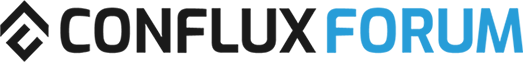# The beavers' guide to calculating block rewards when staking in the PoS Finality Chain

So you want to learn how to calculate the PoS block validation rewards (staking rewards) when staking in the PoS Finality Chain?

Staking rewards depend on:

• Circulating Suppy (`C`): Total circulating supply of CFX. This can be obtained from sources such as CoinMarketCap.
• Staked Supply (`S`): Total amount of staked CFX by all validators. This quantity must be estimated. A good back-of-the-napkin estimate is to sum all of the staked supply from public pools.
• Base Interest Rate (`i`): Fixed base interest rate, fixed at 4%.
• Staked CFX (`staked-CFX`): The amount of CFX that you stake.

You can estimate the staking rewards in the PoS Finality Ledger, with the following formula:

`PoS Interest Rate = sqrt(C / S) * i`
`Annual Staking Profit = staked-CFX * PoS Interest Rate`

Let’s do a test run!

Say there’s a circulating supply of 1.43B (at the time of this post) and there’s currently 50,000,0000 CFX staked across all nodes.

Our `PoS Interest Rate` would be:
`PoS Interest Rate = sqrt(1,430,000,000 / 100,000,000) * 0.04)`, or `0.1513`.

This means that we would get a 15.1261% of interest rate per each staked CFX.

Now, let’s say that we want to stake 10,000 CFX. Our annualized staking profit would be `Annualized Staking Profit = 10,000 * 0.1513`, or `151.2614` CFX.

As you can see, this a slightly more complex mechanism to estimate staking rewards than the governance site, but can yield greater profits. I hope this helps out!

3 Likes

Please recheck your result. 10000*0.1512= 1512,614not 151,2614